# Sudoku Solver — with source code — Fun project — Easiest Way

So guys, in today’s blog we will see how we can implement Sudoku Solver using Computer Vision and Image Processing techniques. So without any further due.

# Let’s do it…

## Code for Sudoku Solver…

`import cv2import imutilsfrom imutils.perspective import four_point_transformfrom skimage.segmentation import clear_borderfrom tensorflow.keras.models import load_modelimport numpy as npfrom Sudoku import solveSudokufrom tensorflow.keras.preprocessing.image import img_to_arrayimport matplotlib.pyplot as plt# Sudoku Solvermodel = load_model('model/model_mnist/')img_path = 'sudoku images/6.png'img_shape = [28,28]def find_puzzle(img):    real = img.copy()    gray = cv2.cvtColor(img,cv2.COLOR_BGR2GRAY)    blur = cv2.GaussianBlur(gray,(7,7),1)        thresh = cv2.adaptiveThreshold(blur,255,cv2.ADAPTIVE_THRESH_GAUSSIAN_C,cv2.THRESH_BINARY,11,2)    thresh = cv2.bitwise_not(thresh)    cnts = cv2.findContours(thresh.copy(),cv2.RETR_EXTERNAL,cv2.CHAIN_APPROX_SIMPLE)    cnts = imutils.grab_contours(cnts)    cnts = sorted(cnts,key=cv2.contourArea,reverse=True)    puzzle_cnt = None    for c in cnts:        peri = cv2.arcLength(c,True)        approx = cv2.approxPolyDP(c,0.02*peri,True)        if len(approx)==4:            puzzle_cnt=approx            break    if puzzle_cnt is None:        raise Exception(("Could not find Sudoku puzzle outline.Try debugging your thresholding and contour steps."))    cv2.drawContours(real, [puzzle_cnt], -1, (0, 255, 0), 2)    puzzle = four_point_transform(img, puzzle_cnt.reshape(4, 2))    warped = four_point_transform(gray, puzzle_cnt.reshape(4, 2))    return puzzle,warpeddef extract_digit(cell):    thresh = cv2.threshold(cell,0,255,cv2.THRESH_BINARY_INV|cv2.THRESH_OTSU)    thresh = clear_border(thresh) #just clear the extra white pixels along the border    cnts = cv2.findContours(thresh.copy(), cv2.RETR_EXTERNAL,cv2.CHAIN_APPROX_SIMPLE)    cnts = imutils.grab_contours(cnts)    # if no contours were found than this is an empty cell    if len(cnts) == 0:return None    # otherwise, find the largest contour in the cell and create a mask for the contour    c = max(cnts, key=cv2.contourArea)    mask = np.zeros(thresh.shape, dtype="uint8")    cv2.drawContours(mask, [c], -1, 255, -1)    (h, w) = thresh.shape    percentFilled = cv2.countNonZero(mask) / float(w * h)    # if less than 3% of the mask is filled then we are looking at noise and can safely ignore the contour    if percentFilled < 0.03:return None    # apply the mask to the thresholded cell    digit = cv2.bitwise_and(thresh, thresh, mask=mask)    kernel = np.ones((1,1),np.uint8)    digit = cv2.dilate(digit,kernel,iterations=1)    # return the digit to the calling function    return digitdef display_numbers_on_board(board,puzzle):    x = puzzle.copy()    k = 0    for i in range(9):        for j in range(9):            startX,startY,endX,endY = cell_locs[k]            testX = int((endX - startX) * 0.33)            testY = int((endY - startY) * -0.2)            testX += startX            testY += endY            cv2.putText(x,str(board[i][j]),(testX,testY),cv2.FONT_HERSHEY_SIMPLEX,0.9,(0,0,255),2)            k+=1    plt.figure(figsize=(10,8))    plt.imshow(x)    plt.xticks([])    plt.yticks([])    plt.show()    return ximg = cv2.imread(img_path)img = imutils.resize(img,width=600)puzzle,warped = find_puzzle(img)puzzle = imutils.resize(puzzle,width=600)warped = imutils.resize(warped,width=600)step_x = warped.shape//9step_y = warped.shape//9board = np.zeros(shape=(9,9),dtype='int')cell_locs = []for i in range(9):    for j in range(9):        topleftx = j*step_x        toplefty = i*step_y        rightendx= (j+1)*step_x        rightendy = (i+1)*step_y        cell = warped[toplefty:rightendy, topleftx:rightendx]        digit = extract_digit(cell)        if digit is not None:            roi = cv2.resize(digit,tuple(img_shape))            roi = roi.astype('float')/255.0            roi = img_to_array(roi)            roi = np.expand_dims(roi,axis=0)            pred = model.predict(roi).argmax(axis=1)            board[i,j] = pred        cell_locs.append([topleftx,toplefty,rightendx,rightendy])        _=display_numbers_on_board(board,puzzle)while 1:    res = input('Are all numbers predicted correctly? (y/n)')    if res=='n':        cx,cy,ele = input('Input row no, col no, correct element of cell For eg. --> 1 2 1:  ').split()        try:            board[int(cx),int(cy)] = int(ele)        except:            print('out of range...')        _ = display_numbers_on_board(board,puzzle)    elif res=='y':        break    else:        print('Wrong choice!!!')    solved = solveSudoku(board)x = display_numbers_on_board(board,puzzle)cv2.imshow('solved',x)cv2.waitKey(0)cv2.destroyAllWindows()`
• Line 1–10 — Importing libraries required for Sudoku Solver.
• Line 12–14 — Load mnist model and the sudoku image. Also declare a constant img_shape which will be the size of our sudoku image after resizing.
• Line 16–45 — This function will find the puzzle in the image through Image Processing techniques.
• Read the image, convert it to grayscale, and then apply Gaussian Blur to remove Gaussian Noise.
• The threshold the image and inverse it. Means convert white to black and black to white.
• Then find the contours and extract the biggest contour in the image. This is probably our sudoku.
• Apply four point transformation to get the straight view of the puzzle and return it.
• Line 49–77 — This function will extract the digit cell from the puzzle and return it so that the mnist model could identify the number in it.
• Line 80–97 — This function will simply put the numbers on the image/board.
• Line 99–100 — Simply read and resize the image.
• Line 102–104 — Extract the puzzle and resize it.
• Line 106–107 — This will tell us the step size we have to take in the x and y-direction. This step is basically the cell size in the x and y directions respectively.
• Line 109 — Create the board with all values initialized with 0s.
• Line 110 — Create array cell_locs which will contain the cell locations/coordinates.
• Line 112–127 — Traverse in the 9*9 loop where the first 9 represents the 9 rows of the board and the second 9 represents the 9 columns of the board. On traversing it will extract the cell images, predict the number using mnist and put that number in the board array we created in Line 109. Also, we will keep on storing the cell locations in the cell_loc array.
• Line 133–145 — If by chance any of the numbers is wrongly predicted by the mnist, correct it.

NOTE — The numbers in blue are predicted numbers for that cell. The text in red is the address of that cell. If any number is wrongly classified by mnist, correct that. Like the first wrong classified number is at (4,7). The original number there is 1 but the mnist said it is 7 so we will correct it first.

• Line 147 — Solve the sudoku using the solveSudoku function that we imported from Sudoku.py. This Sudoku.py is also written by me and I have used Backtracking for solving sudoku.
• Line 149–150 — Display the solved sudoku numbers on the final image and display it.
• Line 152–153 — Destroy all windows.

# Final result of Sudoku Solver…

Do let me know if there’s any query regarding the Sudoku Solver by contacting me on email or LinkedIn. You can also comment down below for any queries.

To explore more Machine Learning, Deep Learning, Computer Vision, NLP, Flask Projects visit my blog — Machine Learning Projects

For further code explanation and source code visit here — https://machinelearningprojects.net/sudoku-solver/

So this is all for this blog folks, thanks for reading it and I hope you are taking something with you after reading this and till the next time 👋…

Read my previous post: HUMAN SEGMENTATION USING U-NET

--

--

--

## More from MLearning.ai

Data Scientists must think like an artist when finding a solution when creating a piece of code. ⚪️ Artists enjoy working on interesting problems, even if there is no obvious answer ⚪️ linktr.ee/mlearning 🔵 Follow to join our 18K+ Unique DAILY Readers 🟠

## Find All Anagrams in a String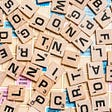## Creating Collections Strapi Backend## Meet the team: Design and Photography## Service objects in Rails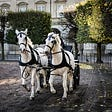## Windows Networking Troubleshooting 7: Network Connectivity Debugging (TrackNblOwner Principle)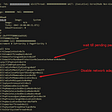## Azure Data Factory: Handle Dynamic Column Names With Rule-Based Mapping In Data Flow## JIRA project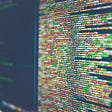## Spring Framework Fundamentals in Examples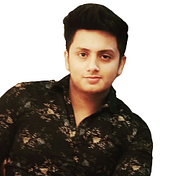## Abhishek Sharma

Data Scientist || Blogger || machinelearningprojects.net

## NEURAL STYLE TRANSFER — WITH SOURCE CODE — EASIEST EXPLANATION — FUN PROJECT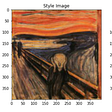## Deep Learning in Medicine: Identifying Pneumonia from a Chest-X-Ray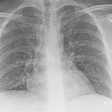## Fix Attribute Error and Runtime Error in tensorflow and keras | Beginner Must Read## More is not always good — Eye disease classification